## Explanation for the Objecttypes used in the NewtonPhysics plugin

While the Newton Game Dynamics library doesn't use patches, the plugin must build hulls for object, they are to be used in the simulation process . This can only work , if you give the plugin the needed information . For this purpose is the "Use as" propertie . To control , if the created collision hull matching the model surface , you can use the propertie "Create model for bounding hull". All "Static" can be explained as deflector object, all "Dynamic" as actor objects .
Some words about the movement for "Dynamic Objects" .
Models with this propertie are animated, i.e. they interact with all other models, they have "Use in simulation" set to "ON" and are subject to the force of gravity. If the model has groups beginning with "Newton _" or "Explode_", then a compound convex hull for these groups model is produced, otherwise a convex hull for the entire model. An example of the compound convex hull is to be seen with the example chain. Only for these objects after the simulation new keyframes (position/rotation) are created. In addition for "Dynamic Objects" an initial impulse (direction/speed/rotation) is computed and used, if keyframes for this model are present. On the basis of the starting time examined whether a following or preceding keyframe is accomplished is set and the computation from this. This does not apply to "Dynamic Explodeobject". Another important thing for "Dynamic Objects" , the center of mass for this models are always at 0,0,0 in the local coordinate system . Other than the rigid body constraint , I don't change position for the masterbone from the model .

Static Object
This object is not movable and it fit always the surface from the A:M model . It affects the dynamic object's, but is not affected self from them . It is created at the position/rotation/scaling/poses at the simulation starttime in the choreographie .

Static with Action
This is a special case for Static Objects and should be used for actors (like the troll or vase project in the samples) . It affects the dynamic object's, but is not affected self from them.
Why I have included this type ? I think this way is more efficient, then to build for each bone from a actor his own collission hull (depends on the cp's the bone is assigned to) and then trace all movements from the bones and translate this movements to forces. And this ways also doesn't considered pose or relationship actions (the next problem, they can change the modelsurface) .
The plugin create the collission hull for this type in two ways , and handle them different .
First case
the model has NO "Newton_" or "Explode_" groups
The collision hull is build at simulation starttime in the same way as a normal static object, means the objecthull fits the modelsurface, but ONLY at simulation starttime
If the model is moved/rotated in the simulationtime , then the created collision hull is also moved/rotated to the correspondending position through the plugin , but the surface is not changed
Second case
the model has "Newton_" or "Explode_" groups
The collision hull for this model is than build as a compound convex hull, where the single convex hulls are created from each group,those the name convention corresponds. At each simulationstep the hull is new created, so the collision hull matches everytime the model surface . This process can slowdown the simulation , so use it with care .
A tip for performance improve
You don't need making "Newton_" groups for all cp's , only the patches they are needed, should have such a group .A look into the vase example project should describe this better, than I can explain .

Dynamic Objects
The plugin handle this object type in two ways .
First way
the model has NO "Newton_" or "Explode_" groups
Here the collision hull is created as a convex hull around the entirely model.
Second way
the model has "Newton_" or "Explode_" groups
Here the collision hull is created as a compound convex hull , based on the groups , they are matching the naming convention .

The difference between this two ways, you can see in the pictures better
 A:M model convex hull (no "Newton_" or "Explode_" groups in the model) compound convex hull (model with "Newton_" or "Explode_" groups)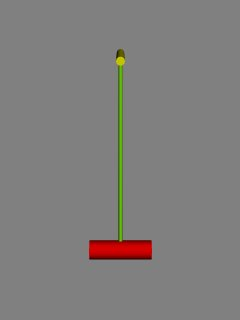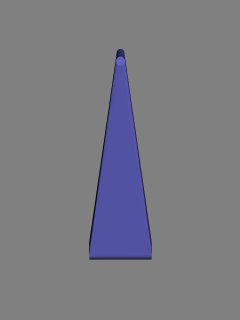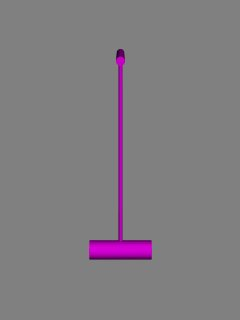The needed "Newton_" groups are different colored in the A:M model

The "Newton_" or "Explode_" groups has also another function , with the density for the individual groups. You can move the center of mass for the model .Todo this , the propertie "use group density" must be set to ON .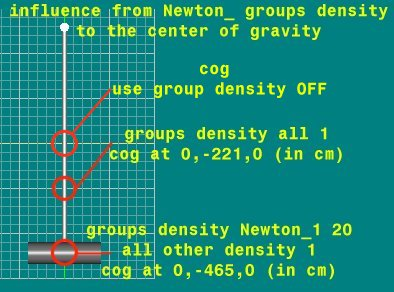If the propertie "use group density" is set to OFF (the default) , then the center of mass is at the center of the axis aligned bounding box around the whole model.

It is also important for Dynamic Objects, Dynamic Boxobjects and Dynamic Sphereobjects , how you have modelled them . For this objecttypes , the center of gravity is always at X/Y/Z 0/0/0 in the modelling window . The only exception is , when you use the group density , then the calculation is shown above .
Here are a little example for this behavior, to view the difference in the movement/rotation in the simulation observe this movie
The black circle is X/Y/Z 0/0/0 in the modelling window .
 Model centered at 0,0,0 Model moved top Model moved down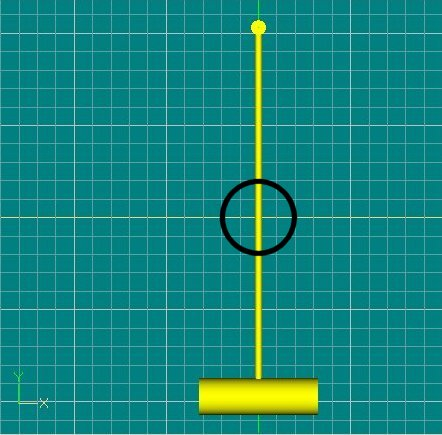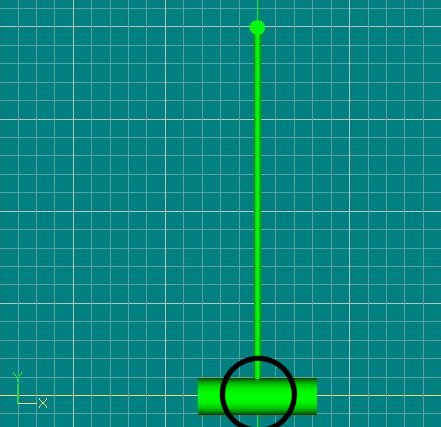Dynamic Boxobject
This is a special case for Dynamic Objects . When you have select this type , always a minimum bounding box around the entirely model is created . This is usefull as example for bricks or dominos . Using this objecttype instead of Dynamic Object increases the simulation speed .

Dynamic Sphereobject
This is a special case for Dynamic Objects . When you have select this type , always a minimum bounding sphere around the entirely model is created . This is usefull as example for spheres :-) . Using this objecttype instead of Dynamic Object increases the simulation speed .

Dynamic Explodeobject
This models are created with the E_Rebuild plugin . For such a model each "Explode_" group is handled as a seperate model, getting their own collision hull and moves independently in the simulation .

Dynamic Breakable Explodeobject
The same as a Dynamic Explodeobject, but they have at the simulation starttime breakable joints between the groups. So are effects possible like the vase example .

Static Fluidsurface
It should be a flat plane (grid resolution 1x1 is enough,(at this time:-)) , but must have the size you need . As example (I assume you use the normal earth gravity), this object has a size from x 1000cm , z 1000 cm, the normaldirection points in y+ , it lies at x,y,z 0 in the choregraphie, and has a deep from 1m, than all objects inside (and also partly inside) the boundingbox (all in cm) x500,y0,z500 and x-500,y-1000,z-500 are reacting in the simulation with the fluid, completly outside this boundingbox they reacting only with the normal gravity given for the object . The deep is calculated in the negated direction of the normals from this object .A example for using as watersurface you can view here. So be sure, in which direction your normals pointing.
When you turn the normaldirection in the example above to y- , you can simulate air (example here) , then the boundingbox for the fluid is x500,y1000,z500 and x-500,y0,z-500 .
How to make a boat stable and swimming , you can read here .

Back